# A radioactive substance has a half-life of 17.33 years. Use the formula n(t) = n0e^ −kt...

A radioactive substance has a half-life of 17.33 years. Use the formula n(t) = n0e^

−kt which tells
you how much is left at time t > 0 to find how much of a 20-gm sample would remain one hundred
years after it is collected. Round your answer to the nearest two decimal places.

t 1/2 = 17.33 yrs ,

k = ln 2 / t1/2 = ( 0.693) / ( 17.33 yrs) = 0.04 yr-1

now no = 20 g , t = 100 yrs , we will find n(t)

n(t) = 20g exp ( - 0.04 yr-1 x 100 yrs)

= 20g exp ( -4)

= 0.37 g

#### Earn Coin

Coins can be redeemed for fabulous gifts.

Similar Homework Help Questions
• ### A scientist begins with 250 grams of a radioactive substance. After 250 minutes, the sample has...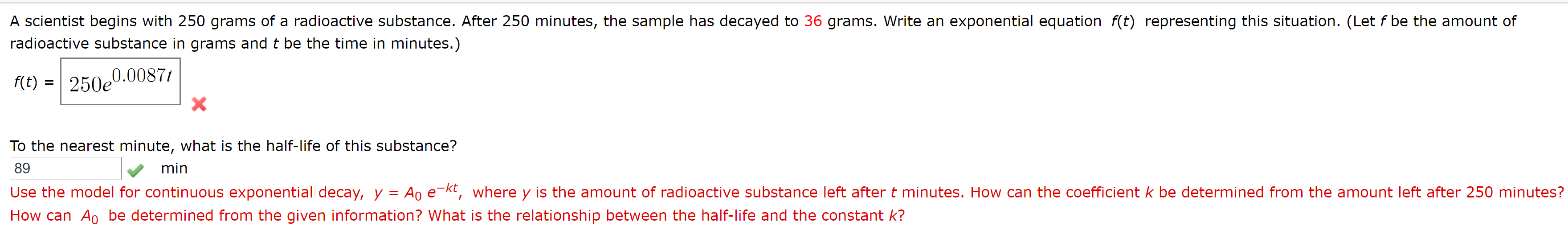A scientist begins with 250 grams of a radioactive substance. After 250 minutes, the sample has decayed to 36 grams. Write an exponential equation f(t) representing this situation. (Let f be the amount of radioactive substance in grams and t be the time in minutes.) f(t) 250e 0.0087t x To the nearest minute, what is the half-life of this substance? 89 min Use the model for continuous exponential decay, y = Ao e-kt, where y is the amount of radioactive...

• ### The half-life of a substance is the time it takes for half of the substance to remain after natural decay

The half-life of a substance is the time it takes for half of the substance to remain after natural decay. Radioactive water (tritium) has a half-life of 12.6 years. How long will it take for 85% of a sample to decay?

• ### The number of grams A of a certain radioactive substance present at time, in years from...

The number of grams A of a certain radioactive substance present at time, in years from the present, t is given by the formula A=45e^-0.0045t What is initial amount of this substance What is half-life of this substance How much will be around in 2500 years

• ### 9. [-14 Points] DETAILS SPRECALC7 4.6.017. This exercise uses the radioactive decay model. The half-life of...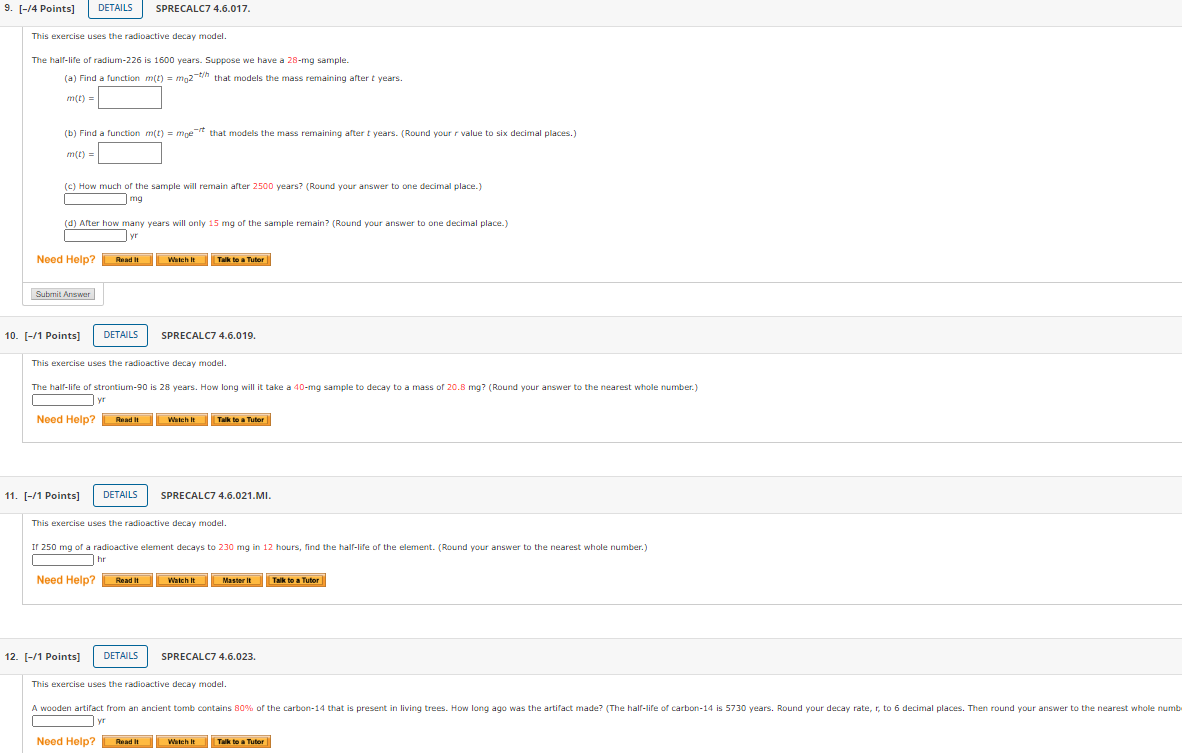9. [-14 Points] DETAILS SPRECALC7 4.6.017. This exercise uses the radioactive decay model. The half-life of radium-226 is 1600 years. Suppose we have a 28-mg sample. (a) Find a function m(t) = moz-th that models the mass remaining after t years. m(t) = (b) Find a function m(t) = moet that models the mass remaining after t years. (Round your value to six decimal places.) m(L) = (c) How much of the sample will remain after 2500 years? (Round your...

• ### A specific radioactive substance follows a continuous exponential decay mode. It has a half-life of 16...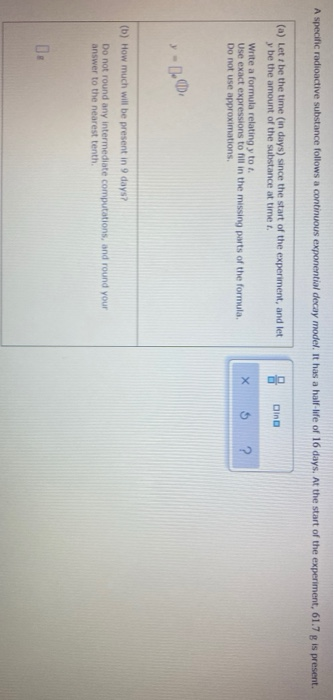A specific radioactive substance follows a continuous exponential decay mode. It has a half-life of 16 days. At the start of the experiment, 61.7 g is present. Din (a) Lett be the time (in days) since the start of the experiment, and let y be the amount of the substance at time. Write a formula relating y toi. Use exact expressions to fill in the missing parts of the formula Do not use approximations X ? (b) How much will...

• ### need help with these two questions. Question 5 (1 point) Willmonite-35 is a newly found radioactive substance with...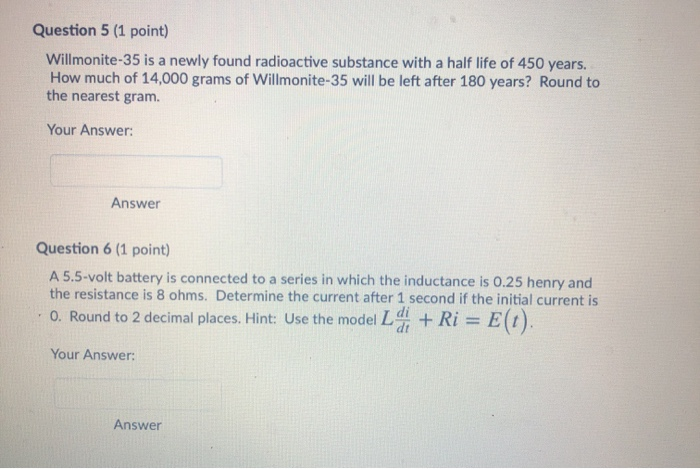need help with these two questions. Question 5 (1 point) Willmonite-35 is a newly found radioactive substance with a half life of 450 years. How much of 14,000 grams of Willmonite-35 will be left after 180 years? Round to the nearest gram. Your Answer: Answer Question 6 (1 point) A 5.5-volt battery is connected to a series in which the inductance is 0.25 henry and the resistance is 8 ohms. Determine the current after 1 second if the initial current...

• ### Radioactive Decay - Half-life and Activity 1 Radioactive decay - Half-life Time 0 1000 21 31...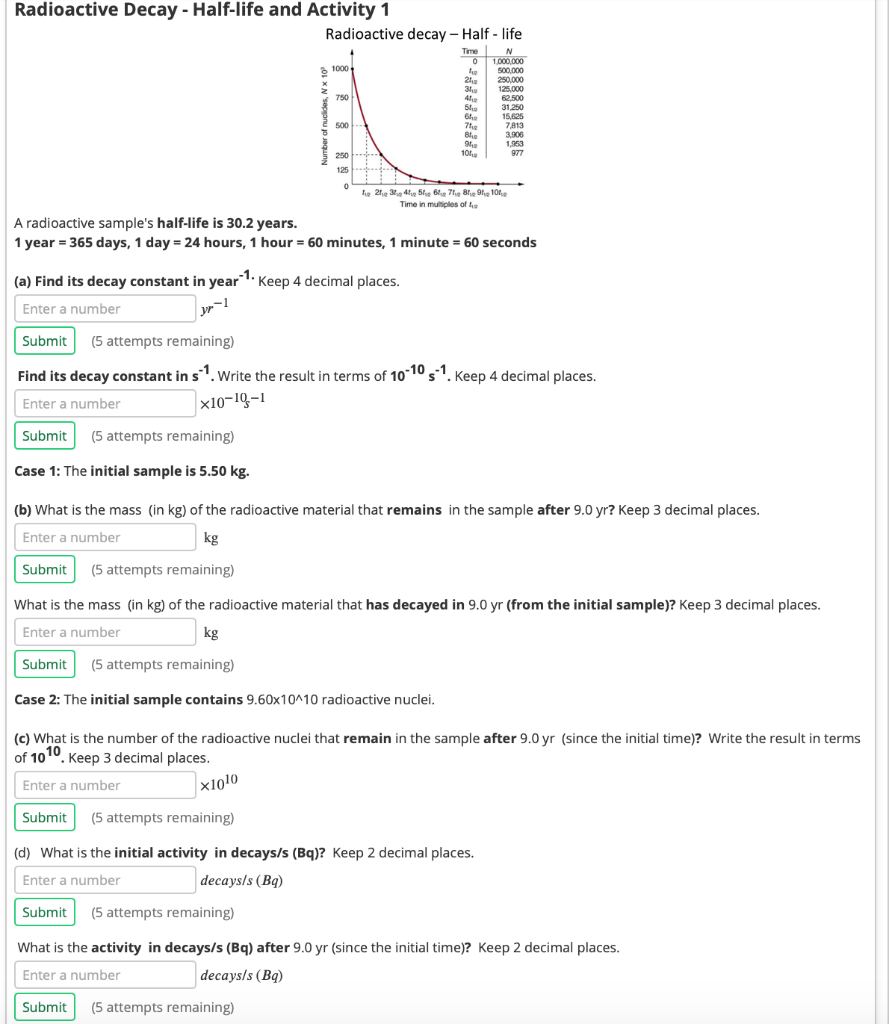Radioactive Decay - Half-life and Activity 1 Radioactive decay - Half-life Time 0 1000 21 31 750 N 1.000.000 500,000 250,000 125,000 62,500 31.250 15.625 7813 3.506 1.953 977 51 6 500 7 BI . 101 250 125 0 tie 21.234.41516171819, 1012 Time in multiples of A radioactive sample's half-life is 30.2 years. 1 year = 365 days, 1 day = 24 hours, 1 hour - 60 minutes, 1 minute = 60 seconds (a) Find its decay constant in year...

• ### How long will it take a sample of radioactive substance to decay to half of its...

How long will it take a sample of radioactive substance to decay to half of its original amount, if it decays according to the function A(t) = 200e^-0.131t, where t is the time in years? Roud to the nearest hundredth year.

• ### How much of a radioactive material with a half-life of 12,000 years is left after 36,000 years of decay

How much of a radioactive material with a half-life of 12,000 years is left after 36,000 years of decay?

• ### An unknown radioactive substance has a half-life of 3.20 hours . If 14.8 grams of the substance...

Part B An unknown radioactive substance has a half-life of 3.20 hours . If 14.8 grams of the substance is currently present, what mass of Ao was present 8.00 hoursago?Part C Americium-241 is used in some smoke detectors. It is an alpha emitter with a half-life of 432 years . How long will it take in years for 43.0% of an Am-241sample to decay?

Free Homework App